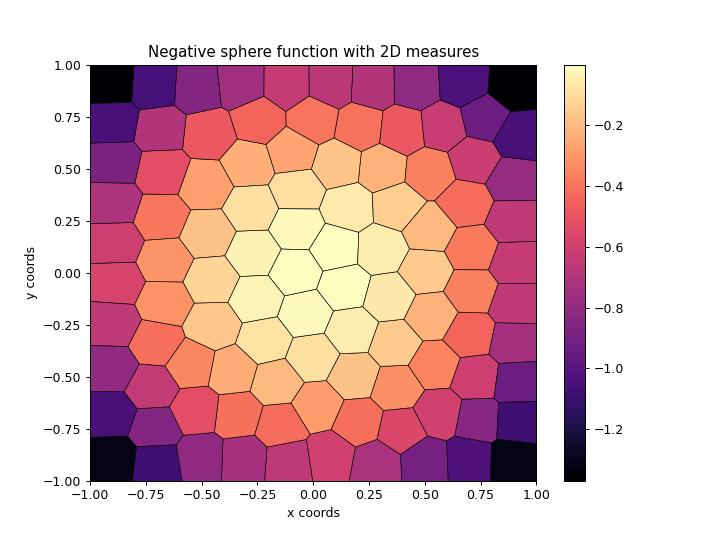# ribs.visualize.cvt_archive_heatmap¶

ribs.visualize.cvt_archive_heatmap(archive, ax=None, plot_centroids=True, plot_samples=False, transpose_bcs=False, cmap='magma', square=False, ms=1, lw=0.5, vmin=None, vmax=None)[source]

Plots heatmap of a CVTArchive with 2D behavior space.

Essentially, we create a Voronoi diagram and shade in each cell with a color corresponding to the objective value of that cell’s elite.

Depending on how many bins are in the archive, ms and lw may need to be tuned. If there are too many bins, the Voronoi diagram and centroid markers will make the entire image appear black. In that case, try turning off the centroids with plot_centroids=False or even removing the lines completely with lw=0.

Examples

>>> import numpy as np
>>> import matplotlib.pyplot as plt
>>> from ribs.archives import CVTArchive
>>> from ribs.visualize import cvt_archive_heatmap
>>> # Populate the archive with the negative sphere function.
>>> archive = CVTArchive(100, [(-1, 1), (-1, 1)])
>>> archive.initialize(solution_dim=2)
>>> for x in np.linspace(-1, 1, 100):
...     for y in np.linspace(-1, 1, 100):
...                     objective_value=-(x**2 + y**2),
...                     behavior_values=np.array([x,y]))
>>> # Plot a heatmap of the archive.
>>> plt.figure(figsize=(8, 6))
>>> cvt_archive_heatmap(archive)
>>> plt.title("Negative sphere function")
>>> plt.xlabel("x coords")
>>> plt.ylabel("y coords")
>>> plt.show()Parameters
• archive (CVTArchive) – A 2D CVTArchive.

• ax (matplotlib.axes.Axes) – Axes on which to plot the heatmap. If None, the current axis will be used.

• plot_centroids (bool) – Whether to plot the cluster centroids.

• plot_samples (bool) – Whether to plot the samples used when generating the clusters.

• transpose_bcs (bool) – By default, the first BC in the archive will appear along the x-axis, and the second will be along the y-axis. To switch this (i.e. to transpose the axes), set this to True.

• cmap (str, list, matplotlib.colors.Colormap) – Colormap to use when plotting intensity. Either the name of a colormap, a list of RGB or RGBA colors (i.e. an Nx3 or Nx4 array), or a colormap object.

• square (bool) – If True, set the axes aspect ratio to be “equal”.

• ms (float) – Marker size for both centroids and samples.

• lw (float) – Line width when plotting the voronoi diagram.

• vmin (float) – Minimum objective value to use in the plot. If None, the minimum objective value in the archive is used.

• vmax (float) – Maximum objective value to use in the plot. If None, the maximum objective value in the archive is used.

Raises

ValueError – The archive is not 2D.